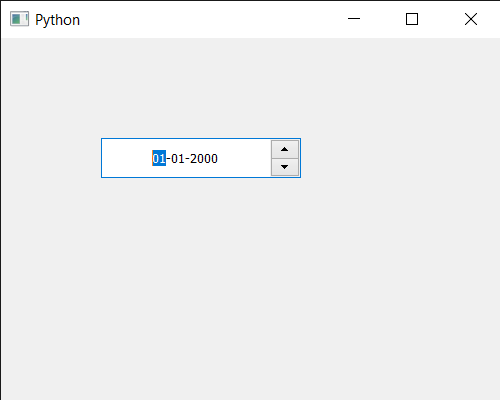Related Articles
PyQt5 QDateEdit – Setting Maximum Time which can be set
• Last Updated : 07 Jul, 2020

In this article we will see how we can set maximum time to the QDateEdit. Sometimes there is a need to set maximum time so that user can’t enter/store time which are beyond the maximum time. We can set time to date edit with the help of `setTime` method.

In order to do this we use `setMaximumTime` method with the QDateEdit object

Syntax : date.setMaximumTime(time)

Argument : It takes QTime object as argument

Return : It returns None

Below is the implementation

 `# importing libraries``from` `PyQt5.QtWidgets ``import` `*` `from` `PyQt5 ``import` `QtCore, QtGui``from` `PyQt5.QtGui ``import` `*` `from` `PyQt5.QtCore ``import` `*` `import` `sys`` ` ` ` `class` `Window(QMainWindow):`` ` `    ``def` `__init__(``self``):``        ``super``().__init__()`` ` `        ``# setting title``        ``self``.setWindowTitle(``"Python "``)`` ` `        ``# setting geometry``        ``self``.setGeometry(``100``, ``100``, ``500``, ``400``)`` ` `        ``# calling method``        ``self``.UiComponents()`` ` `        ``# showing all the widgets``        ``self``.show()`` ` `    ``# method for components``    ``def` `UiComponents(``self``):`` ` `        ``# creating a QDateEdit widget``        ``date ``=` `QDateEdit(``self``)`` ` `        ``# setting geometry of the date edit``        ``date.setGeometry(``100``, ``100``, ``200``, ``40``)`` ` `        ``# alignment``        ``a_flag ``=` `Qt.AlignCenter`` ` `        ``# setting alignment of date``        ``date.setAlignment(a_flag)`` ` `        ``# QTime object``        ``t ``=` `QTime(``10``, ``30``)`` ` `        ``# setting maximum time``        ``date.setMaximumTime(t)``         ` ` ` ` ` `# create pyqt5 app``App ``=` `QApplication(sys.argv)`` ` `# create the instance of our Window``window ``=` `Window()`` ` `# start the app``sys.exit(App.``exec``())`

Output :Attention geek! Strengthen your foundations with the Python Programming Foundation Course and learn the basics.

To begin with, your interview preparations Enhance your Data Structures concepts with the Python DS Course.

My Personal Notes arrow_drop_up